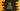# Kotlin program to create a simple calculator## Kotlin program to create a simple calculator:

In this post, we will learn how to create a simple calculator program in Kotlin. We will write a program that will take two numbers as the inputs from the user and perform basic arithmetic operations i.e. sum, subtraction, multiplication, or division based on user input.

I will show you two ways to solve this problem: By using if-else if-else statements or by using when expression.

### Method 1: Kotlin program of a simple calculator by using if-else if-else statements:

Let’s write down a program by using if-else if-else statements:

``````fun main() {
println("Enter the numbers:")
val firstNumber = readLine()?.toDoubleOrNull()
val secondNumber = readLine()?.toDoubleOrNull()

if (firstNumber != null && secondNumber != null) {
println("Enter +, -, * or /:")
val o = readLine().toString()
var result: Double? = null

if (o == '+') {
result = firstNumber + secondNumber
} else if (o == '-') {
result = firstNumber - secondNumber
} else if (o == '*') {
result = firstNumber * secondNumber
} else if (o == '/') {
result = firstNumber / secondNumber
} else {
println("Please enter a valid operator.")
}

if (result != null) {
println("\$firstNumber \$o \$secondNumber = \$result")
}
} else {
print("Please enter valid inputs")
}
}``````

Here,

• The program asks the user to enter the numbers.
• It is using readLine() method to read the user entered value and converts it to a double. These values are stored in the firstNumber and secondNumber variables.
• If the entered numbers are not null, it asks the user to enter the operator symbol. It reads the operator symbol and stores it in the variable o.
• The if, else-if, else blocks checks the value of the variable o and based on its value, it calculates the result.
• The last if block is used to print the final result.

If you run this program, it will print outputs as below:

``````Enter the numbers:
23
33
Enter +, -, * or /:
+
23.0 + 33.0 = 56.0

Enter the numbers:
33
56
Enter +, -, * or /:
*
33.0 * 56.0 = 1848.0

Enter the numbers:
133
4
Enter +, -, * or /:
-
133.0 - 4.0 = 129.0``````

### Method 2: Kotlin program of a simple calculator by using when expression:

The when expression in Kotlin works similar to Java switch statement. We can also use it instead of if-else if-else conditions. Let’s modify the above program to use when expression instead of if-else if-else blocks:

``````fun main() {
println("Enter the numbers:")
val firstNumber = readLine()?.toDoubleOrNull()
val secondNumber = readLine()?.toDoubleOrNull()

if (firstNumber != null && secondNumber != null) {
println("Enter +, -, * or /:")
val o = readLine().toString()
var result: Double? = null

when (o) {
'+' -> {
result = firstNumber + secondNumber
}
'-' -> {
result = firstNumber - secondNumber
}
'*' -> {
result = firstNumber * secondNumber
}
'/' -> {
result = firstNumber / secondNumber
}
else -> {
println("Please enter a valid operator.")
}
}

if (result != null) {
println("\$firstNumber \$o \$secondNumber = \$result")
}
} else {
print("Please enter valid inputs")
}
}``````

It will print similar results.

``````Enter the numbers:
123
23
Enter +, -, * or /:
+
123.0 + 23.0 = 146.0

Enter the numbers:
55
99
Enter +, -, * or /:
*
55.0 * 99.0 = 5445.0``````